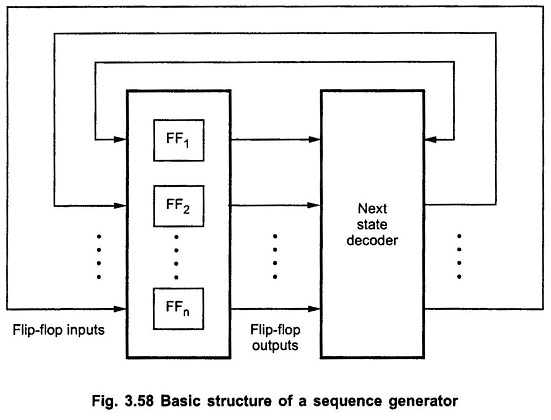## Sequence Generator in Digital Electronics:

A Sequence Generator in Digital Electronics which generates a prescribed sequence of bits, in synchronism with a clock, is referred to as a sequence generator. Fig. 3.58 shows the basic structure of a sequence generator using counters.For the design of sequence generator we must determine the required number of flip-flops and the logic circuit for next state decoder.

#### Design Procedure for the Design of a Clocked Sequential Circuits:

1. It is necessary to first obtain the state table from the given circuit information such as a state diagram, a timing-diagram, or other pertinent information.

2. The number of states may be reduced by state reduction technique if the sequential circuit can be categorized by input-output relationships independent of the number of states.

3. Assign binary values to each state in the state table.

4.Determine the number of flip-flops needed and assign a letter symbol to each.

5. Choose the type of flip-flop to be used.

6. From the state table, derive the circuit excitation and output tables.

7. Using the K-map or any other simplification method, derive the circuit output functions and the flip-flop input functions.

8. Draw the logic diagram

Scroll to Top